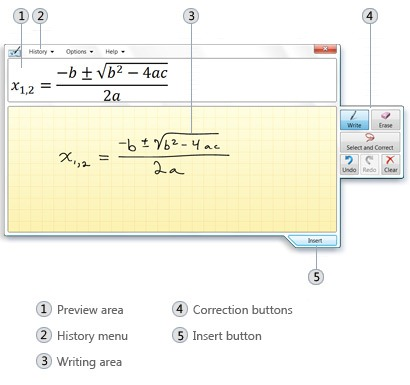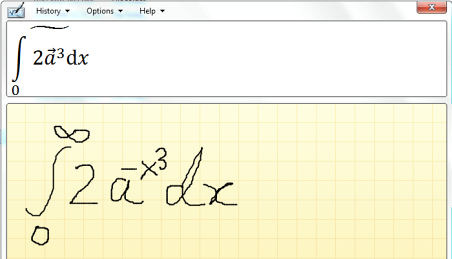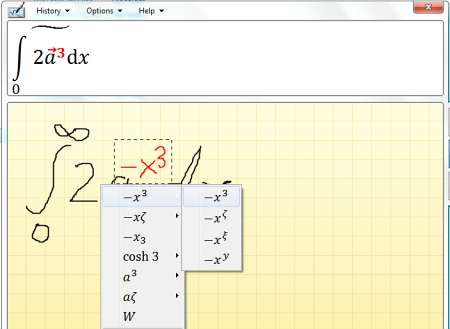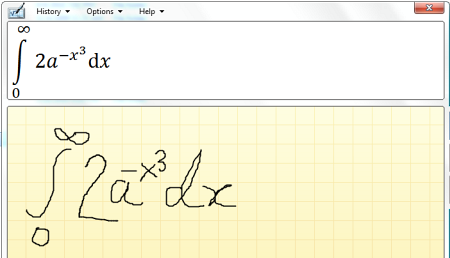# Math Input Panel identifies hand written math equations and symbols

Math Input Panel is a utility found in select Windows 7 editions (Home Premium, Professional, Ultimate and Enterprise) that has the ability to identify mathematical symbols and equations entered or rather drawn onscreen using a mouse, table pen, or a finger (in the case of touch screens).

Math Input Panel uses the math recognizer that’s built into Windows 7 to recognize handwritten math expressions. The recognized expressions are then converted to Mathematical Markup Language (MathML), which is a W3C recommended application of XML for describing mathematical notations. You can then insert the expression into a word-processing or any supported program.Math Input Panel recognizes high school and college-level math. This includes the following:

• Numbers and letters
• Arithmetic
• Calculus
• Functions
• Sets
• Set theory
• Algebra
• Combinatorics
• Probability and statistics
• Geometry
• Vectors and 3D analytic geometry
• Mathematical logic
• Axioms, theorems, and definitions
• Applied mathematics

### How to use Math Input Panel

Open the Start menu and in the search box, type Math Input Panel. Open the program from the list of results. With the mouse or tablet pen, write a math expression in the writing area as clearly as you can. The recognized math will be shown in the preview area.

The recognition engine is pretty good, but not perfect. It requires a little help and coaxing from the user. It is not likely the program will get it right the first time, as you can see from the screen grab below.When this happens, there is a correction tool using which you can select the correct math symbol from a menu.After a couple of corrections, finally the program got it right.Now to insert the expression into a program, click on the program to active it and then use the Insert button at the bottom of the Math Input Panel.

Like I said, you can insert math expressions generated by Math Input Panel into only those programs that support MathML. Major office products such as OpenOffice.org, KOffice, and MS Office 2007 and 2010 editions support MathML. Web browsers that support MathML include Firefox, Chrome, Opera and Safari. Support for Internet Explorer can be added by installing the MathPlayer plugin. Some LaTex editors also support MathML.

1.2.3.